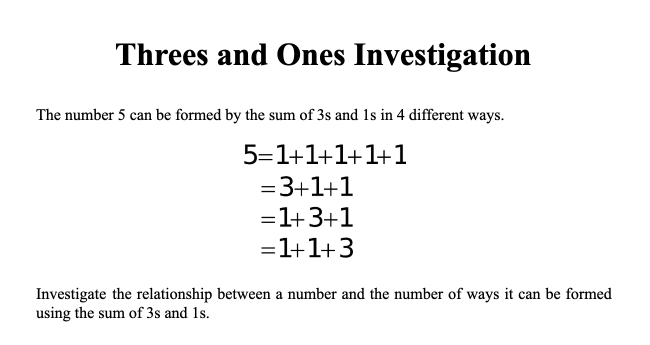# Threes and Ones

Preview:Students investigate the relationship between a number and the number of ways it can be formed using the sum of 3s and 1s.

Project
Big Idea:
Grade 4: Development of computational fluency and multiplicative thinking requires analysis of patterns and relations in multiplication and division.
Curricular Competency:
Reasoning and analyzing: Use reasoning to explore and make connections
Reasoning and analyzing: Develop mental math strategies and abilities to make sense of quantities
Understanding and solving: Develop, demonstrate, and apply mathematical understanding through play, inquiry, and problem solving
Communicating and representing: Communicate mathematical thinking in many ways
Communicating and representing: Explain and justify mathematical ideas and decisions